# Scattering of Microparticles

The following article is from The Great Soviet Encyclopedia (1979). It might be outdated or ideologically biased.

## Scattering of Microparticles

the process of the collision of particles as a result of which the momenta of the particles change (elastic scattering), or, in addition to the change in momenta, either the internal states of the particles also change or other particles are formed (inelastic scattering).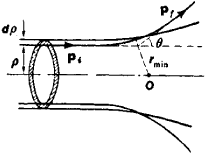Figure 1

One of the basic quantitative characteristics of both elastic scattering and inelastic processes is the effective cross section of the process, usually called simply the cross section, which is the quantity proportional to the probability of the process and having the dimension of area (cm2). The measurement of the cross sections of processes makes it possible to study the laws of interaction of particles and to investigate particle structure. For example, the classical experiments of E. Rutherford on the scattering of α-particles by atoms established the existence of atomic nuclei; information on the structure of nucleons is obtained from experiments on the scattering of high-energy electrons by protons and neutrons (nucleons); and experiments on the elastic scattering of neutrons and protons by protons make it possible to investigate nuclear forces in detail.

Classical theory of scattering. According to the laws of classical (nonrelativistic) mechanics, the problem of the scattering of two particles of masses m1 and m2 can be reduced to the problem of the scattering of one particle of reduced mass μ = m1m2/(m1 + m2) at a fixed center of force by converting to a center-of-mass system for the colliding particles. In such a system, the center of mass of the particles is at rest; that is, the total momentum of the particles is equal to zero. In a force field with center O, the trajectory of a particle is curved—that is, scattering occurs. The angle between the initial (pi) and final (pf) momenta of the scattered particle is called the scattering angle. The scattering angle θ depends on the interaction between particles and on the impact parameter ρ—the distance that a particle would travel from the center of force if interaction were absent (Figure 1). Classical mechanics establishes the following relation between the impact parameter and the scattering angle: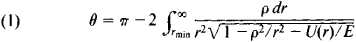where U(r) is the potential energy of interaction, r is the distance to the center of force (rmin is the minimum distance), and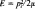is the energy of the particle.

The scattering of an individual particle is usually not measured in an experiment; rather, a beam of identical particles of identical energy is directed toward a target composed of the substance under study, and the number of particles scattered at a given angle is measured. The number of particles dN scattered per unit time at angles lying in the interval θ,θ + dθ is equal to the number of particles passing through a ring 2π pdp per unit time. If η is the flux density of the incident particles (the number of particles passing per unit time through a unit area perpendicular to the direction of motion of the particles in the beam), then dN= 2πρdρ. n, and the elastic scattering cross section is defined as the ratio dN/n and is equal to

(2) dσ = dN/n = 2πρdρ

that is, as noted above, the cross section has the dimension of area. The scattering cross section for all angles—the total scattering cross section—is obtained by integrating equation (2) with respect to all impact parameters. If a is the minimum impact parameter at which θ= 0—that is, the particle passes without deflection—then the total scattering cross section is σ = πa2.

Quantum theory of scattering. In quantum theory, the processes of elastic scattering and inelastic processes are described by scattering amplitudes—complex quantities, the squares of whose moduli are proportional to the cross sections of the corresponding processes. In 1943, W. Heisenberg introduced the scattering matrix, or S-matrix, to describe scattering processes. The matrix elements of the S-matrix determine the amplitudes of various processes. Physical quantities directly measured in experiments, such as the cross section, particle polarization (the average value of the spin operator), and the asymmetry arising on scattering from a polarized target, are expressed in terms of the matrix elements of the S-matrix. On the other hand, the matrix elements of the S-matrix can be calculated if certain assumptions are made regarding the type of interaction. The comparison of experimental results with theoretical predictions makes it possible to test the theory.

The general principles of invariance—invariance with respect to rotations (from which the conservation of angular momentum derives), invariance with respect to reflections (from which the conservation of parity derives), and time reversal —significantly limit the possible type of matrix elements of the S-matrix and make it possible to obtain experimentally verifiable relationships. For example, it follows from the law of conservation of parity that the polarization of a finite particle upon collision of nonpolarized particles is directed along the normal to the scattering plane—the plane passing through the initial and final momenta of the particle. By measuring the direction of the polarization vector, it is possible to determine whether parity is conserved in the interaction responsible for the process. The isotopic invariance of strong interactions leads to relationships between the cross sections of different processes and to the prohibition of certain processes. In particular, it follows from isotopic invariance that on collision of two deuterons, an α-particle and a π0-meson cannot be produced. The investigation of this process experimentally has confirmed the validity of isotopic invariance.

The condition of unitariness of the S-matrix, which follows from the conservation of total probability (the total probability of scattering for all possible modes of disintegration must equal 1), also imposes restrictions on the matrix elements of processes. One of the important relationships that follow from this condition is the optical theorem, which relates the elastic scattering amplitude for an angle of 0° to the total cross section, which is the sum of the elastic scattering cross sections and the cross sections of all possible inelastic processes.

It follows from the general principles of quantum theory, such as the microcausality condition and relativistic invariance, that the matrix elements of the S-matrix are analytic functions in some domains of the complex variables. The analytic properties of the matrix elements of the S-matrix make it possible to obtain a number of relationships between experimentally determined quantities—dispersion relations, the Pomeranchuk theorem, and others.

In the case of elastic scattering of spinless particles, the asymptotic behavior of the wave function Ψ (r), which is a solution of the Schrödinger equation, has the form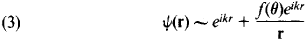Here, r is the distance between particles, k = p/ħ is the wave vector, ρ is the momentum in the center-of-mass system of the colliding particles, ħ is Planck’s constant, θ is the scattering angle, and f(θ) is the scattering amplitude, which depends on the scattering angle and the energy of the colliding particles. The first term in this expression describes free particles with the momentum ρ = ħ k (the incident wave), and the second term describes particles moving from the center (the scattered wave). The differential scattering cross section is defined as the ratio of the number of particles scattered per unit time into the element of the solid angle dΩ to the flux density of the incident particles. The scattering cross section for the angle θ (in the center-of-mass system) over a unit solid angle is equal toFor the scattering amplitude, the following expansion for partial waves (waves with a defined orbital angular momentum l) obtains: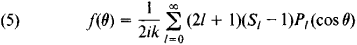Here, pl (cos θ) is a Legendre polynomial and Sl is the expansion coefficient. These depend on the character of the interaction and are the matrix elements of the S-matrix (in the representation for which the S-matrix is diagonal with respect to energy, angular momentum, and the projection of angular momentum). If the number of particles with angular momentum l incident on the center is equal to the number of particles having the same angular momentum moving from the center (the case of elastic scattering), then ǀSlǀ = 1. In the general case, ǀSlǀ ≤ 1. These conditions follow from the condition of unitarity of the S-matrix. If only elastic scattering is possible, then Sl may be represented in the form Sl = e2iδ1, where the δl are real quantities called the scattering phases. If δl = 0 for some l, then scattering to a state with orbital angular momentum l is absent.

The total elastic scattering cross section is equal to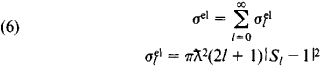whereis the partial elastic scattering cross section for particles with orbital angular momentum l and ƛ = 1/k is the de Broglie wavelength of the particle. When Sl = –1,reaches a maximum and is equal toHere, δl = π/2 (resonance in scattering). Thus, at resonance the cross section of a process is determined by the de Broglie wavelength ƛ; for slow particles (for which ƛ ≫ R0), where R0 is the effective range of forces, the cross section greatly exceeds the quantity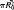(the classical scattering cross section). This fact—incomprehensible from the standpoint of the classical theory of scattering—is a consequence of the wave nature of microparticles.

The behavior of the scattering cross section near resonance is determined by the Breit-Wigner formula: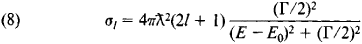where E0 is the energy at which the cross section reaches a maximum (the resonance point) and Γ is the resonance width. When E = E0 ± (½)Γ, the cross section σl is equal to (½)σlmax. The total cross section of all inelastic processes is equal to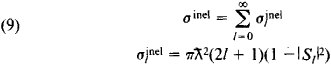The unitary principle restricts the value of the partial cross section for inelastic processes: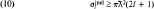For short-range interaction potentials, scattering phases with lbk, where b is the range of forces, play a primary role. This condition may be rewritten as l/kb; the quantity l/k— the impact parameter in quantum theory—determines the minimum distance to which a free particle with an angular momentum l can approach the center of forces. When bk ≪ 1 (low energies), only the S-wave (the partial wave with l = 0) must be taken into account. In this case, the scattering amplitude is equal to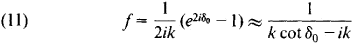and the scattering cross section is independent of the angle (the scattering is spherically symmetrical). At low energies the following expansion holds: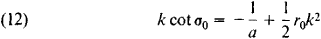The parameters a and r0 are called the scattering length and effective scattering range, respectively. These quantities are determined experimentally and are important characteristics of the forces acting between particles. The scattering length is equal in magnitude and opposite in sign to the scattering amplitude when k = 0. The total scattering cross section at the point k = 0 is equal to σ0 = 4πα2.

If the particles have a bound state with low binding energy, then the scattering of the particles for bk≪ 1 is resonant in character. A typical example is the scattering of neutrons by protons in a state with total spin J = 1; in this state the neutron-proton system has a level corresponding to the bound state—a deuteron. The scattering cross section in this case depends only on the binding energy.

If the parameter bk is small, the scattering phases can be found from experimentally measured values of the cross section and other quantities. This procedure is called phase analysis. The scattering phases found by phase analysis are compared with theoretical predictions and thus make it possible to obtain important information on the character of the interaction.

One of the basic approximate methods of scattering theory is perturbation theory (a solution method based on expansion with respect to a small parameter). If an incident plane wave that describes the initial particles is weakly disturbed by the interaction potential, then Born’s approximation (the first term in the perturbation theory series) is applicable. The elastic scattering amplitude in Born’s approximation is equal to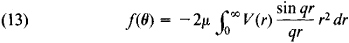where q = 2k sin (Θ/2), V(r) is the interaction potential, and μ = m1m2/(m1 + m2) is the reduced mass (m1 and m2 are the masses of the particles).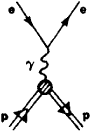Figure 2

The methods of quantum field theory are used to describe scattering processes at high energies. For example, in the lowest order of the perturbation theory, the elastic scattering of electrons (e) by protons (p) is due to the exchange of a photon between the electron and the proton (see the Feynman diagram in Figure 2). The applicability of perturbation theory in this case is based on the smallness of the fine-structure constant α ≈ 1/137 that characterizes the force of the electromagnetic interaction. The electric charge and magnetic form factors of the proton —quantities that characterize the distribution of the electric charge and magnetic moment of the proton (the proton’s electromagnetic structure)—enter the expression for the cross section of this process. Information on these very important characteristics of the proton consequently can be obtained directly from experimentally measured values of the cross section of elastic scattering of electrons by protons. At sufficiently high energies, inelastic processes of particle production become possible in addition to elastic ep scattering. If only electrons are recorded in an experiment, the sum of the cross sections of all possible processes is thus measured.

### REFERENCES

Landau, L. D., and E. M. Lifshits. Kvantovaia mekhanika, 3rd ed. Moscow, 1974. (Teoreticheskaia fizika, vol. 3.)
Davydov, A. S. Kvantovaia mekhanika, 2nd ed. Moscow, 1973.
Goldberger, M., and K. Watson. Teoriia stolknovenii. Moscow, 1967. (Translated from English.)
Mott, N., and H. Massey. Teoriia atomnykh stolknovenii. Moscow, 1951. (Translated from English.)
Sitenko, A. G. Lektsii po teorii rasseianiia. Kiev, 1971.

S. M. BILENKII